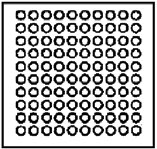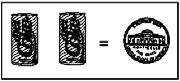Type your answers in the corresponding fields for each question. Click the "Grade Me!" button to see how you did. Use the "Clear" button to start over.

1) How many groups of 10 are there in 250?2) 1 + 2 + 3 + 4 + 5 + 6 + 7 + 8 + 9 + 10 =

3) Two soda cans are worth a nickel. How much are 6 soda cans worth? cents4) How much is half of 7? Write your answer as a fraction. /5) Find the next number in the pattern:  0, 10, 8, 18, 16, 26, 24,